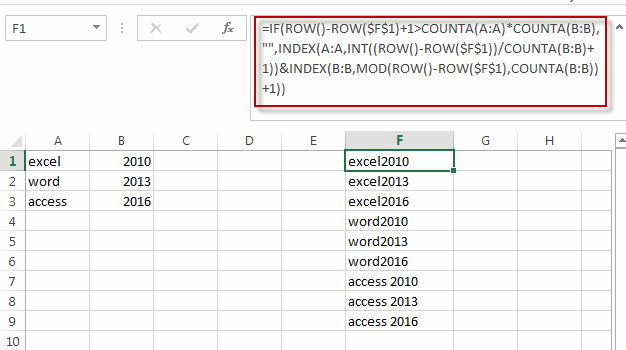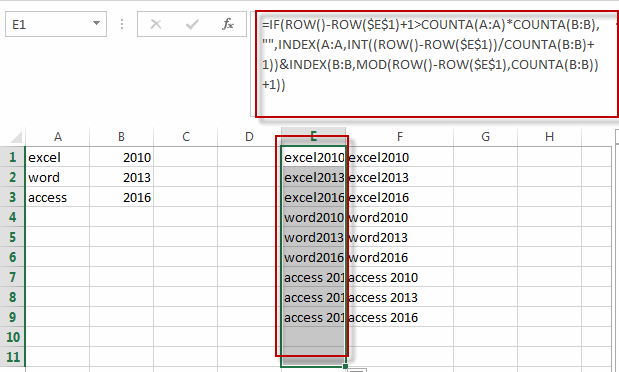# Generate All Possible Combinations of Two Lists in Excel

This post will guide you how to generate all possible combinations with a formula in excel. How do I create list with all possible combinations of two lists in Excel. How to list all combinations from two separate list or range in Excel.

## Generate All Possible Combinations of Two Lists in Excel

Assuming that you have two list of data in different column, A and B, and you want to get a list of all possible combinations from those two list in Column A and B. How to achieve it.

You can use a formula based on the IF function, the ROW function, the COUNTA function, The INDEX function and the MOD function. Like this:

`=IF(ROW()-ROW(\$F\$1)+1>COUNTA(A:A)*COUNTA(B:B),"",INDEX(A:A,INT((ROW()-ROW(\$F\$1))/COUNTA(B:B)+1))&INDEX(B:B,MOD(ROW()-ROW(\$F\$1),COUNTA(B:B))+1))`

Type this formula into Cell F1, and then drag the AutoFill Handle down column F until you get cells that look empty.Note: if you want to use this formula into other cell, you need to change the absolute cell reference to that cell. Such as: form F1 to E1.

`=IF(ROW()-ROW(\$E\$1)+1>COUNTA(A:A)*COUNTA(B:B),"",INDEX(A:A,INT((ROW()-ROW(\$E\$1))/COUNTA(B:B)+1))&INDEX(B:B,MOD(ROW()-ROW(\$E\$1),COUNTA(B:B))+1))`### Related Functions

• Excel INDEX function
The Excel INDEX function returns a value from a table based on the index (row number and column number)The INDEX function is a build-in function in Microsoft Excel and it is categorized as a Lookup and Reference Function.The syntax of the INDEX function is as below:= INDEX (array, row_num,[column_num])…
• Excel ROW function
The Excel ROW function returns the row number of a cell reference.The ROW function is a build-in function in Microsoft Excel and it is categorized as a Lookup and Reference Function.The syntax of the ROW function is as below:= ROW ([reference])….
• Excel IF function
The Excel IF function perform a logical test to return one value if the condition is TRUE and return another value if the condition is FALSE. The IF function is a build-in function in Microsoft Excel and it is categorized as a Logical Function.The syntax of the IF function is as below:= IF (condition, [true_value], [false_value])….
• Excel MOD function
he Excel MOD function returns the remainder of two numbers after division. So you can use the MOD function to get the remainder after a number is divided by a divisor in Excel. The syntax of the MOD function is as below:=MOD (number, divisor)….
• Excel INT function
The Excel INT function returns the integer portion of a given number. And it will rounds a given number down to the nearest integer.The syntax of the INT function is as below:= INT (number)…
• Excel COUNTA function
The Excel COUNTA function counts the number of cells that are not empty in a range. The syntax of the COUNTA function is as below:= COUNTA(value1, [value2],…)…

Related Posts

Creating a Table with Automatic Row Numbering

When creating an Excel Table, you may use a calculation relying on the ROW function to insert row numbers automatically. The formula in A2, which has been copied down, is as follows: =ROW()-ROW(Table1[#Headers]) Note: the default table name is Table1. ...

Get Address of First Cell in Range

We will learn how to get address of first cell in range in this post. At Instance, we must determine the address of first cell in range to use as the data table's value. For the DATA table, we have ...

Abbreviate Names Or Words in Excel

As an MS Excel user, you might have come across a task where you need to abbreviate different names or words, and there are also possibilities that you might have done this task manually by assuming that there isn't any ...

Filter or Extract with a Partial Match

Assume you have a table consisting of a few cells having few values, and you want to filter out the set of records with the partial match. You might take it easy and would prefer to manually filter out the ...

Extract matching values From Two Lists

Suppose that you are working with two lists containing few values, and you want to extract the matching values from those two lists into another separate list. You might prefer to manually extract the matching values from the two lists, ...

Extract all Partial Matches

Just assume that you have a range of data that contains a text string and you want to extract the few partial matches into another separate range of cells; then you might think that it's not a big deal; because ...

Extract Multiple Match Values into Separate Columns

If you have a few values/items in the excel sheet and you are thinking that with the aid of the “VlOOKUP” function you can look for a specific value, extract it and then put the matching item into the separate ...

Average the Last N Numeric Values in Excel

AVERAGE function is one of the most popular functions in Excel. Apply AVERAGE together with some other functions, we can calculate average simply for some complex situations. In this article, we will introduce you to calculate average of the last ...

How To Sum the Largest N Values in Excel

Sometimes we may want to sum the largest N numbers or top N numbers in a range. In this article, we will show you the method of “SUM the Largest N Numbers” by a simple formula which consist of SUMPRODUCT ...

How to Sum the Smallest N Values in Excel

Sometimes we may want to sum the first smallest N numbers in a range in Excel. In this article, we will show you the method of “SUM the Smallest N Numbers” by a simple formula which consist of SUMPRODUCT and ...

Sidebar